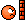# Need Help With Arg() Function

3 replies to this topic

### #1far2055

far2055

•• Members
•• 67 posts
• Gender:Male
• Location:Iran
• Interests:Robotics - Programming - Mathematics - Physics - Computer <br />ANN ( Artificial Neural Network )<br />QBasic Lover-->before-->now-&gt;*CPLua&quot; Lover.

• Calculators:

Posted 18 February 2008 - 02:04 PM

I am working on a Contol ToolBox for ClassPad as I said before. I use CPBasic. I have some problem with arg() function. Check this:
```define f(x)=(25)/( (x*j)^2 + 4*(x*j) + 25 )
done
arg( f( 1 ) )
-0.17
arg( f( x ) ) | x = 1
where j is imaginary unit.

### #2Stiftblei

Stiftblei

Newbie

•• Members
•• 3 posts

• Calculators:

Posted 18 February 2008 - 03:11 PM

Which OS do you have? OS3 gives me this:

### #3Debb

Debb

Newbie

•• Validating
•• 23 posts
• Gender:Female

• Calculators:

Posted 19 February 2008 - 07:08 PM

Hi,

After talking to my math friend, she explained that the ClassPad is evaluating the expression and then substituting the value for x. If you want to force the ClassPad to evaluate using the value for x, enclose the expression and value within parenthesis.

arg(f(x)|x=1)

Debb

I am working on a Contol ToolBox for ClassPad as I said before. I use CPBasic. I have some problem with arg() function. Check this:

```define f(x)=(25)/( (x*j)^2 + 4*(x*j) + 25 )
done
arg( f( 1 ) )
-0.17
arg( f( x ) ) | x = 1
where j is imaginary unit.

### #4far2055

far2055

•• Members
•• 67 posts
• Gender:Male
• Location:Iran
• Interests:Robotics - Programming - Mathematics - Physics - Computer <br />ANN ( Artificial Neural Network )<br />QBasic Lover-->before-->now-&gt;*CPLua&quot; Lover.

• Calculators:

Posted 22 February 2008 - 04:59 PM

Which OS do you have? OS3 gives me this:

I use OS3. I have some problem with the link!

Hi,

After talking to my math friend, she explained that the ClassPad is evaluating the expression and then substituting the value for x. If you want to force the ClassPad to evaluate using the value for x, enclose the expression and value within parenthesis.

arg(f(x)|x=1)

Debb

Thanks for your help. But still there is a problem. What about the 2D plotter??? How can I force it to evaluate my function before evaluating the arg() function?#### 0 user(s) are reading this topic

0 members, 0 guests, 0 anonymous users